GeeksforGeeks App
Open AppBrowser
Continue

# How to find the Surface Area of a Prism?

A prism is a 3-D shape having two identical polygons facing each other. The identical polygons are called the bases of the prism, where they can be triangles, squares, rectangles, pentagons, or any other n-sided polygon. The other faces of a prism are parallelograms or rectangles. A prism can be a regular prism or an irregular prism based on the shape of its base, i.e., whether the base is a regular polygon or an irregular polygon. Also, there are different types of prisms based on the shape of the base of a prism, such as

• Triangular prisms,
• Square prisms,
• Rectangular prisms,
• Pentagonal prisms,
• Hexagonal prisms, etc.

### Surface Area of Prism

The total surface area of a prism is the total area occupied by all its faces. To find out the surface area of a prism, we need to calculate the areas of all of its faces and then add all the areas obtained. The lateral surface area of a prism is the area occupied by the faces of a prism, excluding the identical faces (bases of a prism) facing each other.

Lateral surface area of a prism = Base perimeter × height

Now, the total surface area of a prism is equal to the sum of its lateral surface area and the areas of its two bases.

The general formula for calculating the surface area of any type of prism is:

Total surface area of a Prism = 2(Base Area)+ (Base perimeter × height)

### Triangular prism

A triangular prism is a prism whose bases are triangular. Let “H” be the height of the prism; “a, b, and c” are the lengths of the sides of triangular bases, and “h” be their height.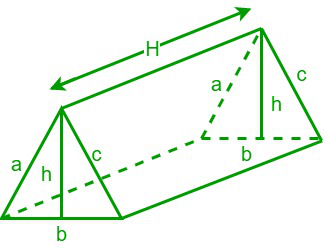Now, lateral surface area of the triangular prism = Base perimeter × height

We know that perimeter of a triangle = Sum of its three sides = a + b + c

Lateral surface area of the triangular prism = (a + b + c) H

Total surface area of a triangular prism = 2(Base Area)+ (Base perimeter × height)

We know that area of a triangle = 1/2 base × height = 1/2 b × h

Total surface area of a triangular prism = 1/2bh + (a + b + c) H

### Square Prism

A square prism is a prism whose bases are squares. Let “a” be the length of the side of a square and “h” be the height of the prism.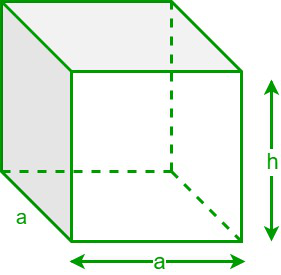Now, lateral surface area of the square prism = Base perimeter × height

We know that perimeter of a square = Sum of its four sides = 4a

Lateral surface area of the square prism = 4ah

Total surface area of a square prism = 2(Base Area)+ (Base perimeter × height)

We know that the area of a square = a2 square units.

Total surface area of a square prism = 2a2 + 4ah

### Rectangular prism

A rectangular prism is a prism whose bases are rectangles. Let “h” be the height of the prism, and “l and b” be the length and breadth of the rectangular bases.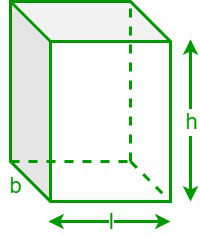Now, lateral surface area of the rectangular prism = Base perimeter × height

We know that perimeter of a rectangle = Sum of its four sides = 2 (l + b)

Lateral surface area of the rectangular prism = 2(l + b)h

Total surface area of a rectangular prism = 2(Base Area)+ (Base perimeter × height)

We know that the area of a rectangle = (l × b) square units.

Total surface area of a rectangular prism = 2lb + 2bh + 2lh

### Pentagonal prism

A pentagonal prism is a prism whose bases are pentagons. Let “h” be the height of the prism, “a” be the apothem length of the prism, and “b” be the base length of the prism.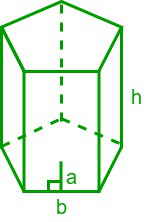Lateral surface area of the pentagonal prism = 5bh

Total surface area of a pentagonal prism = 5ab + 5bh

### Hexagonal Prism

A hexagonal prism is a prism whose bases are hexagons. Let “a” be the length of the side of a hexagon and “h” be the height of the prism.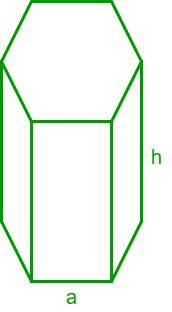Lateral surface area of the hexagonal prism = 6ah

Total surface area of a hexagonal prism = 3√3a2 + 6ah

### Sample Problems

Problem 1: Find the height of the square prism if its total surface area is 58 cm2, and the length of the side of the square base is 2 cm.

Solution:

Given data,

The total surface area of the square prism = 58 cm2

The length of the side of the square base = 2 cm

We know that,

The total surface area of a square prism = 2a2 + 4ah

⇒ 58 = 2 × (2)2 + 4 × 2 × h

⇒ 58 = 8 + 8h

⇒ 50 = 8h

⇒ h = 50/8 = 6.25‬ cm,

Hence, the height of the given prism is 6.25‬ cm.

Problem 2: What is the surface area of a prism whose base area is 15 square units, a base perimeter of 24 units, and its height is 8 units?

Solution:

Given data,

Base area = 15 square units

Base perimeter = 24 units

Height of the prism = 8 units

We have,

The total surface area of the prism = (2 × Base Area) + (Base perimeter × height)

= (2 × 15) + (24 × 8)

= 30 +  192 = 222 square units.

Hence, the surface area of the given prism = 222 square units.

Problem 3: What is the lateral surface area of a triangular prism whose base perimeter is 30 cm and the height of the prism is 12 cm?

Solution:

Given data,

The base perimeter of the prism = 30 cm2

The height of the prism = 12 cm

We know that,

The lateral surface area of the prism = Base perimeter × height

= 30 × 12= 360 sq. cm

Hence, the lateral surface area of the prism is 360 sq. cm.

Problem 4: Find the surface area of the regular hexagonal prism if the height of the prism is 10 in and the length of the side of the base is 7 in.

Solution:

Given data,

The height of the prism (h) = 10 in

The length of the side of the base (a) = 7 in

The surface area of a regular hexagonal prism = 6ah + 3√3a2

= 6 × 7 × 10 + 3√3(7)2

= (420 + 147√3) sq. in

Hence, the surface area of the given prism is (420 + 147√3) sq. in.

Problem 5: Determine the lateral surface area and the total surface of a rectangular prism if the length and breadth of the base are 11 cm and 8 cm, respectively, and the height of the prism is 14 cm.

Solution:

Given data,

The length of the rectangular base (l) = 11 cm

The breadth of the rectangular base (b) = 8 cm

The height of the prism (h) = 14 cm

We have,

The lateral surface area of the prism = Base perimeter × height

= 2 (l + b) h = 2 × (11 + 8) × 14 = 532‬ sq. cm

We know that,

The total surface area of the rectangular prism = 2 (lb + bh + lh)

= 2 × (11 × 8 + 8 × 14 + 11 × 14)

= 2 × (88 + 112 + 154) = 708 sq. cm

Hence, the lateral and total surface areas of the given rectangular prism are 532 sq. cm and 708 sq. cm, respectively.

Problem 6: If the total area of ​​a pentagonal prism is 125 square units and its height and apothem length are 10 units and 6 units respectively, determine its base length.

Solution:

Given data,

The total surface area of the pentagonal prism = 125 square units

The height of the prism (h) = 10 units

Apothem length (a) = 6 units

We know that,

The total surface area of the pentagonal prism = 5ab + 5bh

⇒ 125 = 5b (a+ h)

⇒ 125/5 = b (6 + 10)

⇒ 25 = b × (16) ⇒ b = 25/16 = 1.5625 units

Hence, the base length is 1.5625 units

My Personal Notes arrow_drop_up
Related Tutorials